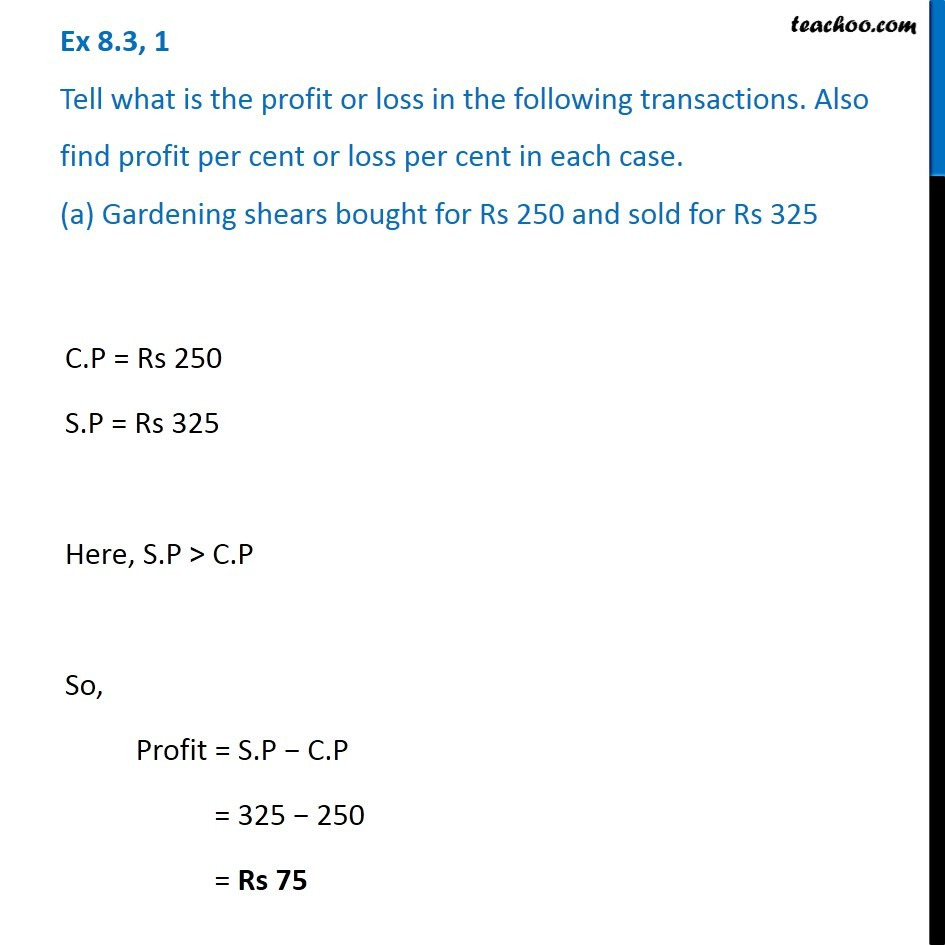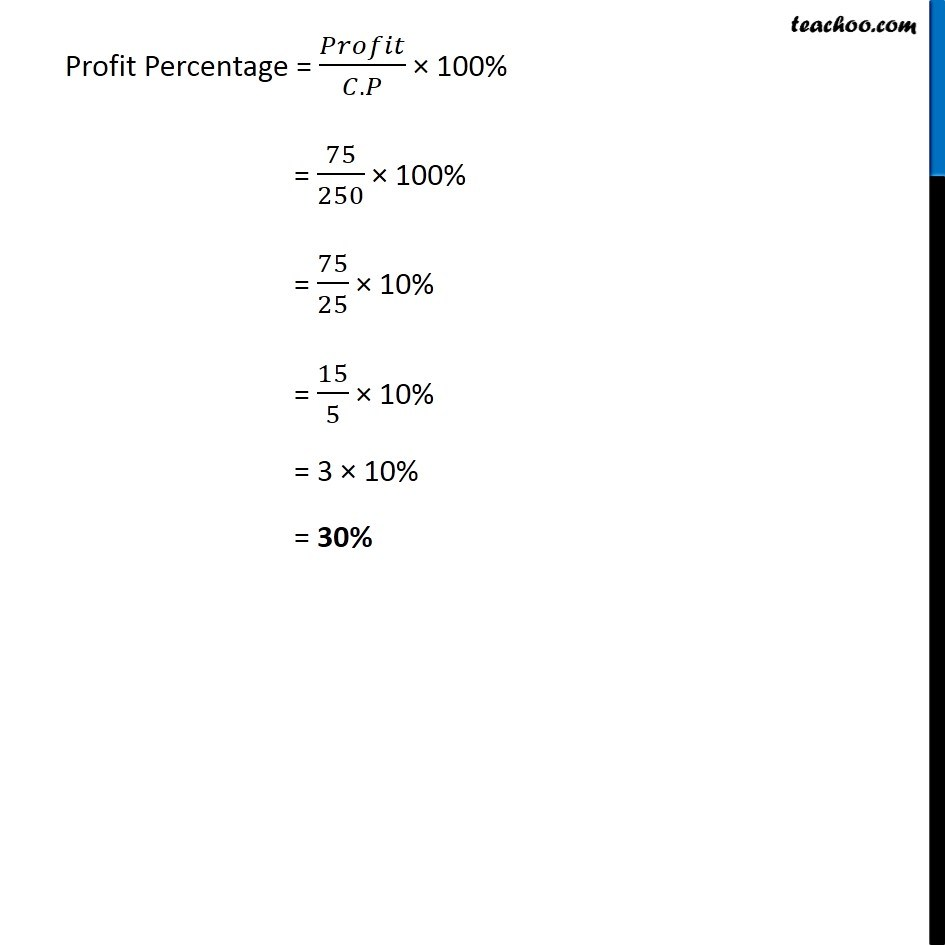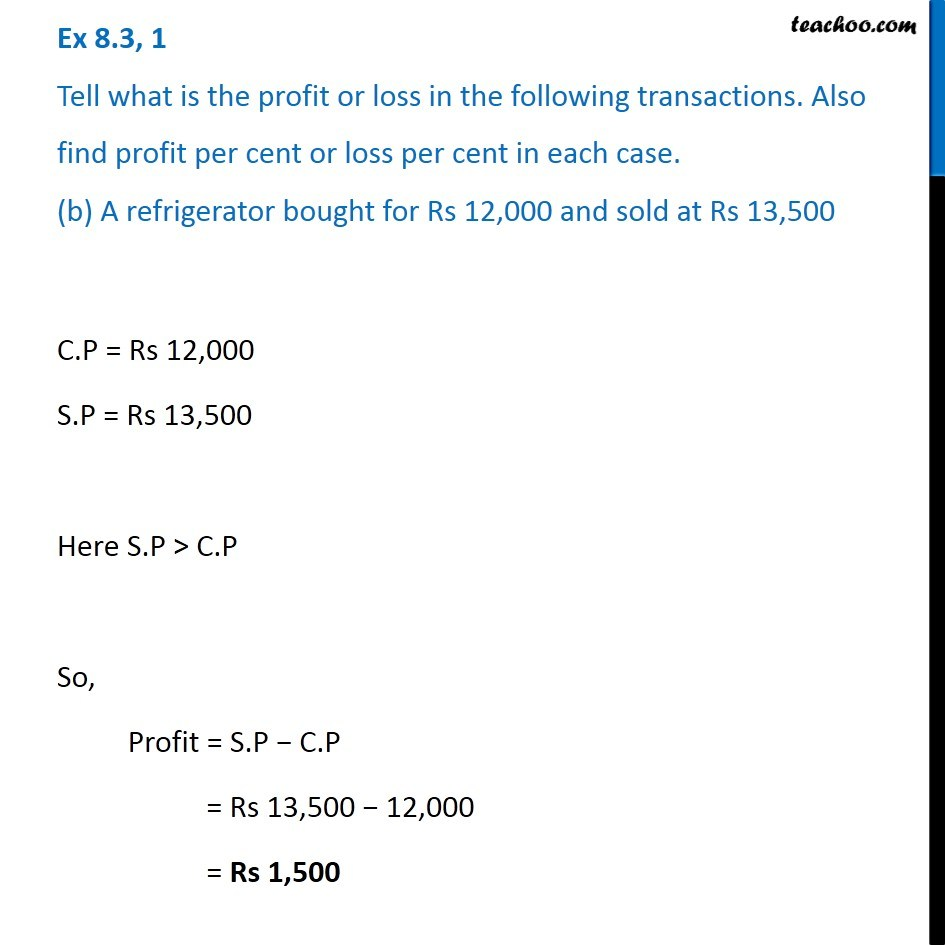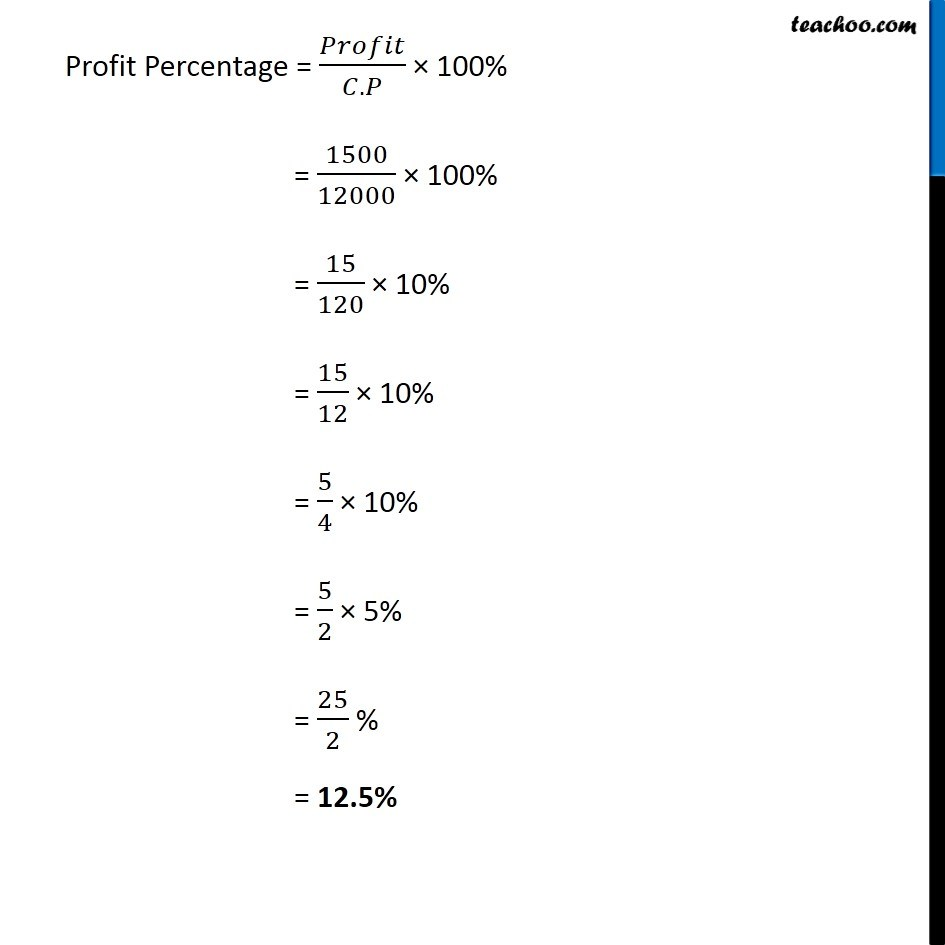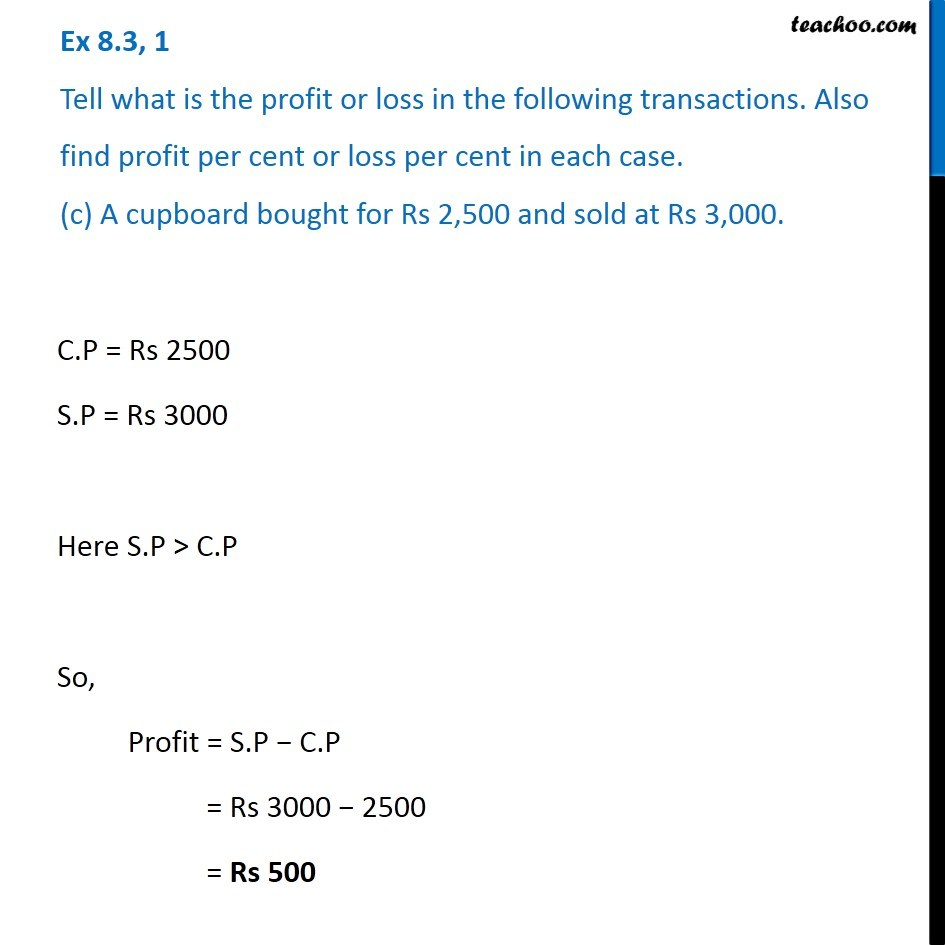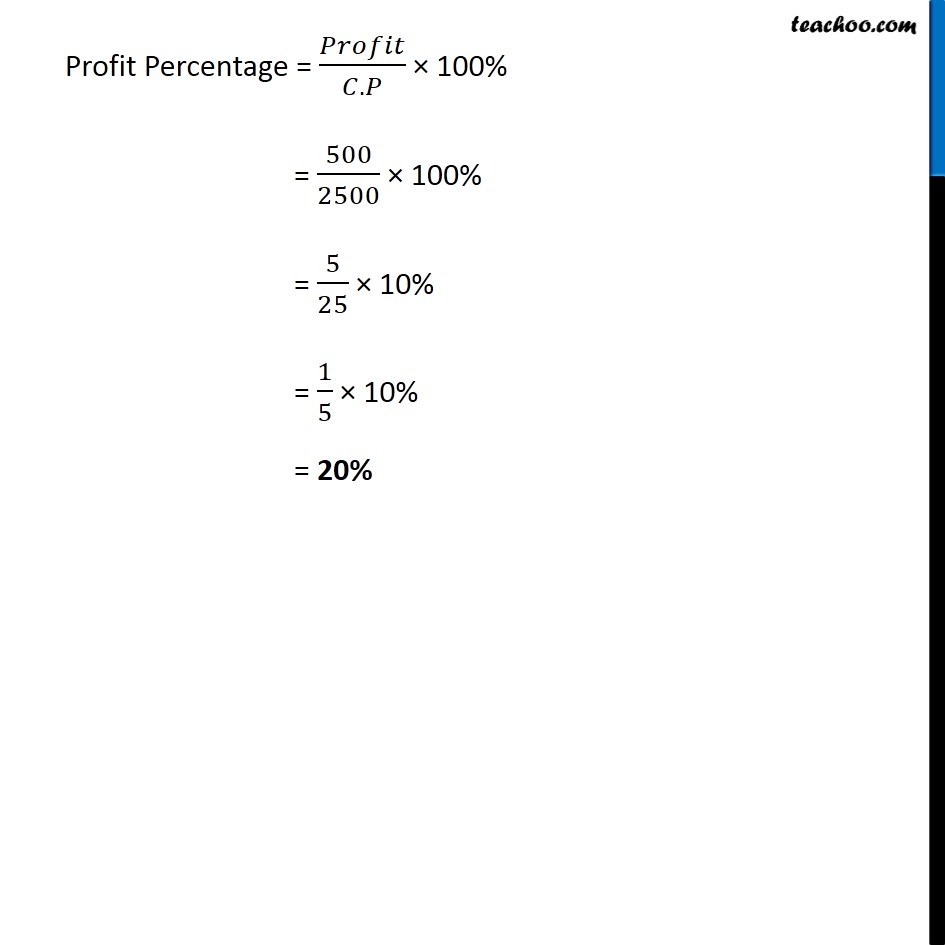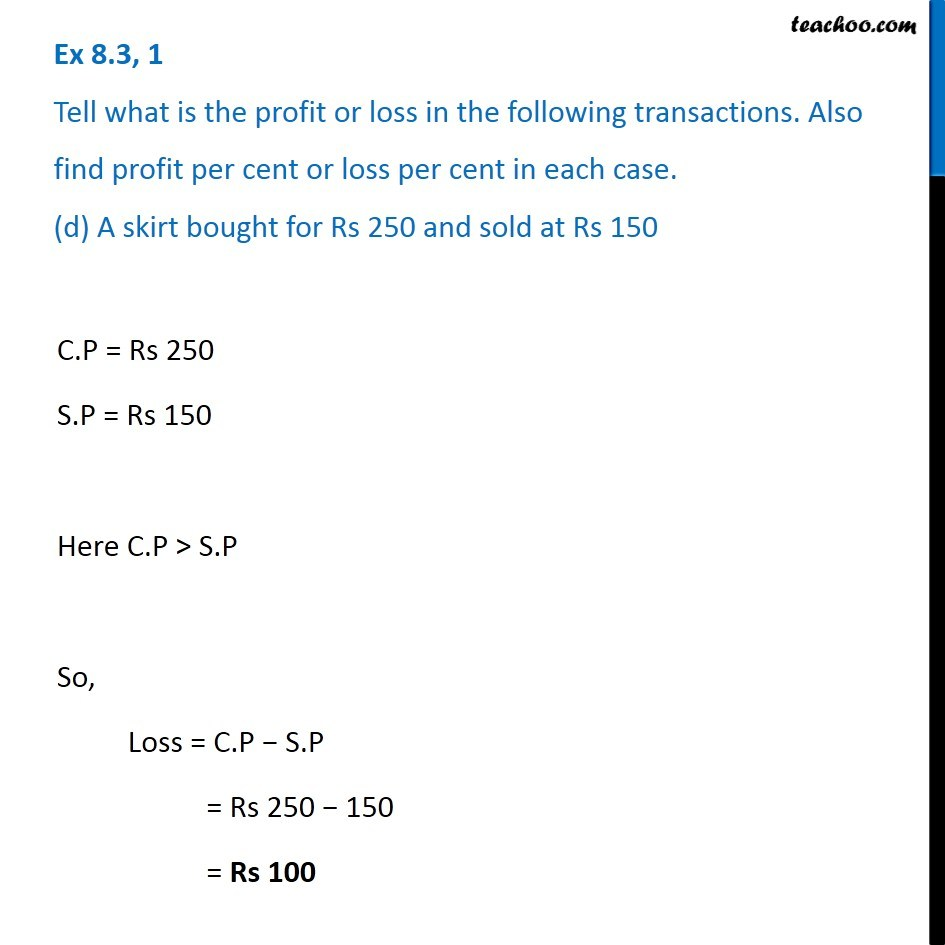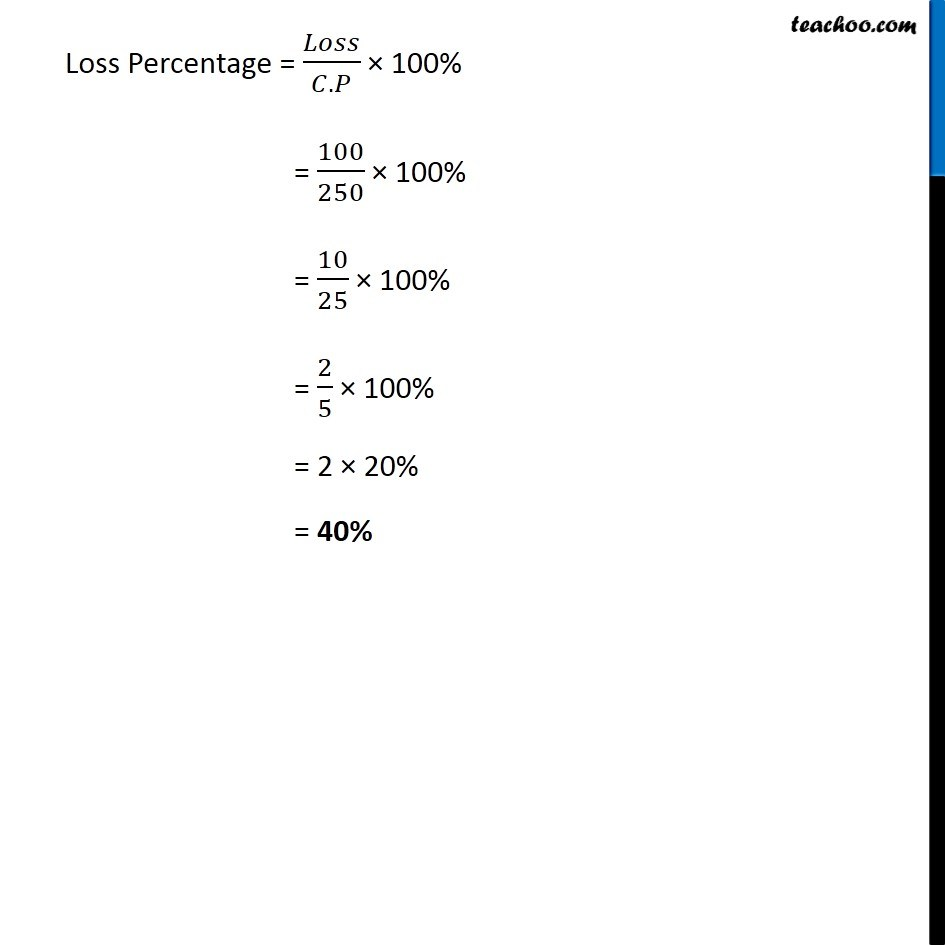Subscribe to our Youtube Channel - https://you.tube/teachoo

1. Chapter 8 Class 7 Comparing Quantities
2. Serial order wise
3. Ex 8.3

Transcript

Ex 8.3, 1 Tell what is the profit or loss in the following transactions. Also find profit per cent or loss per cent in each case. (a) Gardening shears bought for Rs 250 and sold for Rs 325 C.P = Rs 250 S.P = Rs 325 Here, S.P > C.P So, Profit = S.P − C.P = 325 − 250 = Rs 75 Profit Percentage = 𝑃𝑟𝑜𝑓𝑖𝑡/(𝐶.𝑃) × 100% = 75/250 × 100% = 75/25 × 10% = 15/5 × 10% = 3 × 10% = 30% Ex 8.3, 1 Tell what is the profit or loss in the following transactions. Also find profit per cent or loss per cent in each case. (b) A refrigerator bought for Rs 12,000 and sold at Rs 13,500 C.P = Rs 12,000 S.P = Rs 13,500 Here S.P > C.P So, Profit = S.P − C.P = Rs 13,500 − 12,000 = Rs 1,500 Profit Percentage = 𝑃𝑟𝑜𝑓𝑖𝑡/(𝐶.𝑃) × 100% = 1500/12000 × 100% = 15/120 × 10% = 15/12 × 10% = 5/4 × 10% = 5/2 × 5% = 25/2 % = 12.5% Ex 8.3, 1 Tell what is the profit or loss in the following transactions. Also find profit per cent or loss per cent in each case. (c) A cupboard bought for Rs 2,500 and sold at Rs 3,000. C.P = Rs 2500 S.P = Rs 3000 Here S.P > C.P So, Profit = S.P − C.P = Rs 3000 − 2500 = Rs 500 Profit Percentage = 𝑃𝑟𝑜𝑓𝑖𝑡/(𝐶.𝑃) × 100% = 500/2500 × 100% = 5/25 × 10% = 1/5 × 10% = 20% Ex 8.3, 1 Tell what is the profit or loss in the following transactions. Also find profit per cent or loss per cent in each case. (d) A skirt bought for Rs 250 and sold at Rs 150 C.P = Rs 250 S.P = Rs 150 Here C.P > S.P So, Loss = C.P − S.P = Rs 250 − 150 = Rs 100 Loss Percentage = 𝐿𝑜𝑠𝑠/(𝐶.𝑃) × 100% = 100/250 × 100% = 10/25 × 100% = 2/5 × 100% = 2 × 20% = 40%

Ex 8.3